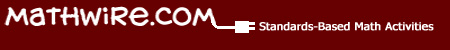Number Sense ActivitiesSome students need prompts to help them write mathematical expressions for target numbers.   Climb the Ladder is an activity that prompts students to move from all addition or subtraction problems and include many mathematical topics to generate equivalent names.   The student starts at the bottom of the ladder and completes the first rung using the prompt provided.   He/she then moves up to the next rung, etc. and works to complete as many rungs as possible in the allotted time.   The teacher may also include a traditional name-collection box for students to use after they reach the top so that they continue to generate equivalent expressions of their choice.   Many students write more diverse mathematical expressions as a result of using this prompted approach.

Suggestions for Using Climb the Ladder:

• Have students write equivalent expressions for the date each day.
• Have students write equivalent expressions for the school day count.
• Roll two dice (or 3-4 dice) to get the day's number.
• Create a bulletin board for 100 and challenge students to find 100 equivalent expressions for 100 to celebrate the 100th day of school.
• Use the open-ended ladder and have students compete in pairs or small groups to generate equivalent mathematical expressions for the given number.   Score the responses in a Scattergorie-type approach.   If anyone else in the group has the same expression, each student crosses out that expression and no one receives points for it.   This approach challenges students to look for more unique mathematical expressions in order to score points for the round.

Templates:   Three different templates are included for Climb the Ladder.   Templates are designed to be used in sheet protectors.   This strategy allows students to use dry-erase markers and re-use the same paper for many Climb the Ladder routines.These problems are designed for students in Grades 3-6. Students should be familiar with theName That Number game and should be comfortable writing mathematical expressions for a target number.